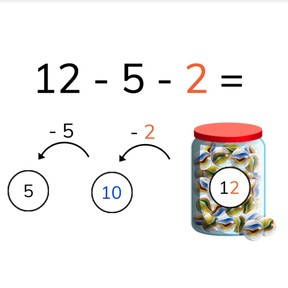Subtraction to 20 using grouping with three numbers

# Subtraction to 20 using grouping with three numbers8,000 schools use Gynzy92,000 teachers use Gynzy1,600,000 students use Gynzy

## General

Students learn to subtract numbers to 20 with three numbers. They learn to first look for the subtraction problem that equals 10, and then to subtract the second number.

1.OA.C

## Relevance

It is important to be able to subtract to 20 with three numbers, so you can determine how many you have left when multiple groups of objects are taken away.

## Introduction

Give a student a box or crate with 10 objects. These could be pencils, erasers, or anything else. You can choose to label the box with 10 to make clear that there are 10 objects in the box. Another student says a number from 1-9. The student with the box then removes that number of objects from the box. How many objects are left in the box? Repeat this with other students (and possibly other boxes with objects). Students then are given subtraction problems with 10.

## Development

Show students the jar of marbles, and say that the jar has 12 marbles. Show the subtraction problem which shows that 5 and 2 marbles are removed from the jar. Explain to students that we are first going to look for the number that, when taken away, equals 10. Show students that if we take 2 away, we have 10 marbles left. Then we take away 5, and find the difference of 5 marbles. Students are next shown a large jar of marbles. First count the marbles in the jar. Then drag the marbles to the correct tray to subtract to 10, and then take away the second number. Practice this again with the eggs, making sure that students know to first subtract the number that will equal 10. Finally students practice with subtraction problems without visual support. In the first exercise, the number which should be subtracted first comes first in the given subtraction problem, in the second set, the number is at different places in the subtraction problem. Remind students that they need to look carefully to determine which number to subtract with to equal 10.

Check that students understand subtraction to 20 using grouping with three numbers by asking the following questions:
- How do you subtract with grouping?
- Which number do you look for first?
- Why is this useful?
- Do you only look at the first number in the subtraction problem? (no, at all to see which, when subtracted, equals 10)

## Guided practice

Students first are given subtraction problems in which the numbers given are in subtraction order, namely with the subtrahend that equals 10 given first. Then students are given subtraction problems in which they must determine the subtraction order.

## Closing

Check that students are able to determine which number to subtract first to equal ten, and then subtract the second number. Emphasize that by finding the number that subtracts to 10, they make the subtraction problem easier and faster to solve. Check that students have understand the instruction by asking how to solve subtraction problems with 3 numbers. Students are then asked to solve a few subtraction problems.
To close, play a game with the students. This can be done as a class, or in groups. A first student is given a number card from 11-20, and they get the title of "Seller". Two other students get a number card from 1-9, and they are the "Buyers". Note Make sure that one of the "Buyer's" number cards is equal to the ones of the "Seller's" card. Example: Seller has 19, one of the buyers must have 9. Students must then determine which "Buyer" goes first to subtract to 10. They then complete the subtraction problem. You can make the agreement as a class that students who have calculated the subtraction problem tell how they solved their problem, and if they get the correct answer, can get new number cards.

## Teaching tips

Students who have difficulty with subtraction to 20 with three numbers can first practice with subtraction to 10. They can do this with support of manipulatives like erasers, markers, or MAB blocks. Students set out the numbers on the table in piles and then take them away from the first number to fid the difference. They can also practice decomposing 10.

## Instruction materials

- Box or tray
- sets of objects in tens (markers, erasers, blocks, etc)
- number cards in set of 1-9 and 11-20

### The online teaching platform for interactive whiteboards and displays in schools

• Save time building lessons

• Manage the classroom more efficiently

• Increase student engagement